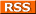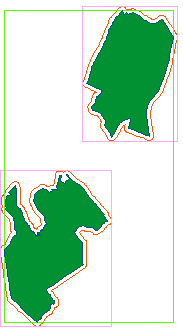Comments Rss
Extent Expand Buffer Distance: PostGIS - ST_Extent, Expand, ST_Buffer, ST_Distance

# Extent Expand Buffer Distance ST_DWithin

In this quick exercise, we will explore the following PostGIS OGC functions: Extent, Expand, Buffer, Distance

## Extent

Extent is an aggregate function - meaning that it is used just as you would use SQL sum, average, min, and max often times in conjunction with group by to consolidate a set of rows into one. Pre-1.2.2 It returned a 2-dimensional bounding box object (BBOX2D) that encompasses the set of geometries you are consolidating.

Unlike most functions in PostGIS, it returns a postgis BBOX object instead of a geometry object.
In some cases, it may be necessary to cast the resulting value to a PostGIS geometry for example if you need to do operations that work on projections etc. Since a bounding box object does not contain projection information, it is best to use the setSRID function as opposed to simply casting it to a geometry if you need projection information. SetSRID will automagically convert a BBOX to a geometry and then stuff in SRID info specified in the function call.

## Extent3d

Extent has a sibling called Extent3d which is also an aggregate function and is exactly like Extent except it returns a 3-dimensional bounding box (BBOX3D).

## ST_Extent

Starting around version 1.2.2, Extent and Extent3d will be deprecated in favor of ST_Extent . ST_Extent will return a BOX3D object.

## Expand (< 1.3.1), ST_Expand (1.2.2 +)

Expand returns a geometry object that is a box encompassing a given geometry. Unlike extent, it is not an aggregate function. It is often used in conjunction with the distance function to do proximity searches because it is less expensive than the distance function alone.

The reason why expand combined with distance is much faster than distance alone is that it can utilize gist indexes since it does compares between boxes so therefore reduces the set of geometries that the distance function needs to check.

Note in versions of PostGIS after 1.2, there exists a new function called ST_DWithin which utilizes indexes, simpler to write than the expand, &&, distance combination.

ST_Distance will be the preferred name in version 1.2.2 and up

The following statements return equivalent values, but the one using Expand is much faster especially when geometries are indexed and commonly used attributes are indexed.

```Find all buildings located within 100 meters of Roslindale
Using distance and Expand: Time with indexed geometries: 14.5 seconds - returns 8432 records
```
FROM neighborhoods n, buildings b
WHERE n.name = 'Roslindale' and expand(n.thegeom_meter, 100) && b.thegeom_meter
and distance(n.thegeom_meter, b.thegeom_meter) < 100
```

Using distance alone: Time with indexed geometries: 8.7 minutes - returns 8432 records
```
FROM neighborhoods n, buildings b
WHERE n.name = 'Roslindale'
and distance(n.thegeom_meter, b.thegeom_meter) < 100
```
```

## ST_DWithin (1.3.1 and above)

We will write the above using the new ST_DWithin to demonstrate how much easier it is.

Using ST_DWithin: Time with indexed geometries: 14.5 seconds - returns 8432 records
```	```
FROM neighborhoods n, buildings b
WHERE n.name = 'Roslindale' and ST_DWithin(n.thegeom_meter, b.thegeom_meter, 100)
```
```

## Within (< 1.3.1), ST_Within (1.3.1 and above)

ST_Within(A,B) returns true if the geometry A is within B. There is an important distinction between Within and ST_Within and that is the ST_Within does an implicit A&&B call to utilize indexes where as Within and _ST_Within do not.

1.3.1 and above do

```	```
FROM neighborhoods n, buildings b
WHERE n.name = 'Roslindale' and ST_Within(b.thegeom_meter, n.thegeom_meter)
```
```

Pre 1.3.1 do

```	```
FROM neighborhoods n, buildings b
WHERE n.name = 'Roslindale' and b.thegeom_meter && n.thegeom_meter AND Within(b.thegeom_meter, n.thegeom_meter)
```
```

## ST_Buffer (+1.2.2), Buffer (< 1.2.2)

Buffer returns a geometry object that is the radial expansion of a geometry expanded by the specified number of units. Calculations are in units of the Spatial Reference System of this Geometry. The optional third parameter sets the number of segments used to approximate a quarter circle (defaults to 8) if third argument is not provided.
This is a much more involved process than the expand function because it needs to look at every point of a geometry whereas the expand function only looks at the bounding box of a geometry.

Aliases: ST_Buffer (MM-SQL)

### Correcting Invalid Geometries with Buffer

Buffer can also be used to correct invalid geometries by smoothing out self-intersections. It doesn't work for all invalid geometries, but works for some. For example the below code will correct invalid neighborhood geometries that can be corrected. Note in here I am also combining the use with MULTI since in this case buffer will return a polygon and our table geometries are stored as multi-polygons. If your column geometry is a POLYGON rather than a MULTIPOLYGON then you can leave out the MULTI part.

``` UPDATE neighborhoods SET the_geom = multi(buffer(the_geom, 0.0)) WHERE isvalid(the_geom) = false AND isvalid(buffer(the_geom, 0.0)) = true ```

## Pictorial View of Buffer, Expand, Extent

In this section we provide a graphical representation of what the operations Buffer, Expand, Extent look like when applied to geometries.

 LegendCorresponding Queries Original: ``````SELECT the_geom, name FROM neighborhoods WHERE name IN('Hyde Park','Roxbury')`````` Expand: Draws a box that extends out 500 units from bounding box of each geometry``````SELECT expand(the_geom, 500) as geom, name FROM neighborhoods WHERE name IN('Hyde Park', 'Roxbury')`````` Extent: Draws a single bounding box around the set of geometries``````SELECT extent(the_geom) as thebbox, name FROM neighborhoods WHERE name IN('Hyde Park', 'Roxbury')`````` Buffer: Extends each geometry out by 500 units. ``````SELECT buffer(the_geom,500) as geom, name FROM neighborhoods WHERE name IN('Hyde Park', 'Roxbury')``````

Post Comments About Extent Expand Buffer Distance: PostGIS - ST_Extent, Expand, ST_Buffer, ST_Distance

This Document is available under the GNU Free Documentation License 1.2 http://www.gnu.org/copyleft/fdl.html & for download at the BostonGIS site http://www.bostongis.com

Boston GIS      Copyright 2023      Paragon Corporation